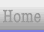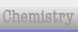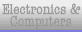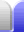# Messing With Infinity

### Overview

Infinity is not really a number, but more of a concept. Because it's not really a number, we have to be careful about how we use it.

When we start to do stuff with infinity, strange things can happen. For example, positive infinity can appear to be the same as negative infinity, as seen in the tangent function at 90°. We can also get very useful results.

### 0.9 ≡ 1

0.9 is a recurring number. The string of 9s carries on forever. Because it never stops, 0.9 is exactly the same as 1. This is also mentioned in the rational numbers section on the Number Sets page.

1/3 = 0.333...

2/3 = 0.666...

3/3 = 0.999...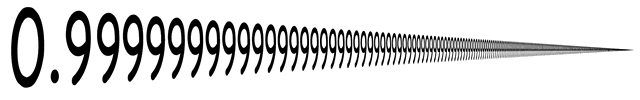∴ 0.999... ≡ 1

### The four values of 0 / 0

The fraction made by zero divided by zero is a curious one. Taking a simplified view of fractions, we appear to have several basics that clash with each other.

• Zero divided by any number is zero.

0 / 0 = 0

• Any number divided by itself is 1 (a unity fraction).

0 / 0 = 1

• Any number divided by zero is infinity.
• (As a fraction's denominator approaches zero the value of the fraction aproaches infinity.)

0 / 0 = ∞

• Zero is neither positive or negative.
• (This means that as a fraction's negative denominator approaches zero the value of the fraction aproaches negative infinity.)

0 / 0 = -∞

This implies that 0 / 0 is 0, 1, ∞, and -∞ all at the same time. It is not possible for a simple numeric fraction to have four values at once.

0 / 0 = undefined

### 0 = ∞ = 1

Let's say we have a number x, such that x is the sum of all natural numbers to infinity.

x = 1 + 2 + 3 + 4 + 5 + ...

Let's add a zero to both sides.

0 + x = x = 0 + 1 + 2 + 3 + 4 + 5 + ...

And now we'll substract the second equation from the first.

x - x = 1 + 1 + 1 + 1 + 1 + ...
⇒ 0 = 1 + 1 + 1 + 1 + 1 + ...
0 = ∞

This itself is nonsensical, because it's saying zero equals infinity, but we'll continue. Let's add a zero to both sides again.

0 = 0 + 1 + 1 + 1 + 1 + 1 + ...

And subtract the last equation from the second to last.

0 - 0 = 1 + 0 + 0 + 0 + 0 + 0 + ...
0 = 1

Hence, zero equals one. Not because it actually does, but because we messed with infinity (by substracting infinity from infinity).Home Astronomy Chemistry Electronics Mathematics Physics Field Trips Turn on javascript for email link